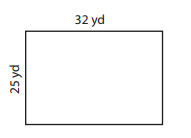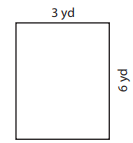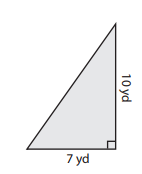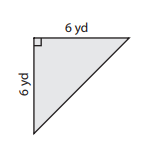# Review GED Math Part 2

In part two of our GED® Math prep, you get familiar with Geometry. Are you ready to test your knowledge?

Take a 10-Question Practice Test.

Question 1 of 10

1. Find the perimeter of this rectangle.Use this formula
P=2l + 2w
l-length
w-width
A.
B.
C.
D.

Question 1 of 10

Question 2 of 10

2. Find the area of this rectangle.Use this formula.

Area = w × h

w = width
h = height

A.
B.
C.
D.

Question 2 of 10

Question 3 of 10

3. Find the area of this triangle.

Use this formula
A = ½ bh
h-height
b-baseA.
B.
C.
D.

Question 3 of 10

Question 4 of 10

4. Find the area of this triangle.

Use this formula
A = ½ bh
h-height
b-baseA.
B.
C.
D.

Question 4 of 10

Question 5 of 10

5. Find the exact circumference of this circle.Use this formula
C = 2πr
or
C = πd;
π ≈ 3.14
d-diameter
A.
B.
C.
D.

Question 5 of 10

Question 6 of 10

6. Find the exact circumference of this circle.Use this formula.
C = 2πr
or
C = πd;
π ≈ 3.14
d-diameter
A.
B.
C.
D.

Question 6 of 10

Question 7 of 10

7. Find the surface area of this rectangular prism.Use this formula.

Surface Area = 2ab + 2bc + 2ac

A.
B.
C.
D.

Question 7 of 10

Question 8 of 10

8. Find the surface area of this rectangular prism.Use this formula.

Surface Area = 2ab + 2bc + 2ac

A.
B.
C.
D.

Question 8 of 10

Question 9 of 10

9. How many degrees form one complete sweep through a circle like noon to midnight on a clock?

A.
B.
C.
D.
E.

Question 9 of 10

Question 10 of 10

10. How many degrees sweep the half circular arc between directly east and directly west?

A.
B.
C.
D.
E.

Question 10 of 10

Next lesson: Commutative Property Continue learning. The online GED Classes that are offered on this website are a part of the Covcel GED® Prep Course. So it seems a good idea for us to give you more information about it. Covcel’s GED Prep Course can help you reach your goals sooner than you ever thought possible. In this way, Covcel is leaps and bounds ahead of its competitors. Covcel GED Prep relies on its principle of “small steps” that helps students achieve short-term goals (learning a particular topic or subject) en route to the ultimate long-term goal (obtaining one’s HSE certificate). Of particular importance is that Covcel’s GED Prep program is entirely student-driven.

In fact, you choose which topics to study—called “cherry-picking”—and you decide how much time to spend studying.

By expertly crafting its materials to instill confidence, and enhance and maintain motivation.

The GED exam contains four separate subtests that cover the academic fields of Social Studies, Mathematics, Science, and Language Arts. In most states, you are allowed a time frame of teo years to complete the entire GED battery. Online GED testing is not an option. Test-takers are required to show up in person at an official GED testing site.

Upgrade to a Full version of GED Prep

Upgrade to the Full Version of GED Prep from Covcel and speed up your learning. Score increase guarantee. Check out the Covcel Review.